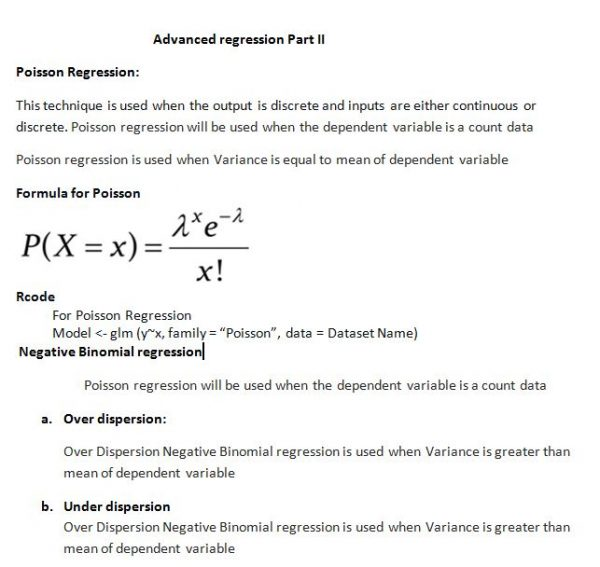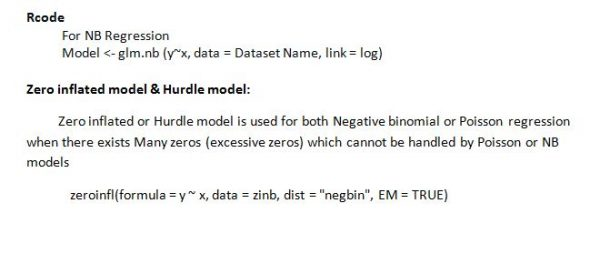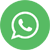Advanced Regression Part 2

# Poisson Regression:

This technique is used when the output is discrete and inputs are either continuous or discrete. Poission regression will be used when the dependent variable is a count data Poisson regression is used when variance is equal to mean of dependent variable

Negative Binomial regression

poission regression will be used when the dependent variable is a count data

a. Overdispersion: Over-Dispersion Negative Binomial regression is used when Variance is greater than the mean of dependent variable

b.Under dispersion: Over Dispersion Negative Binomial regression is used when Variance is greater than the mean of the dependent variable